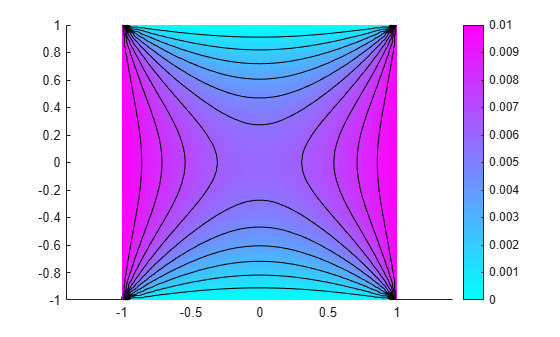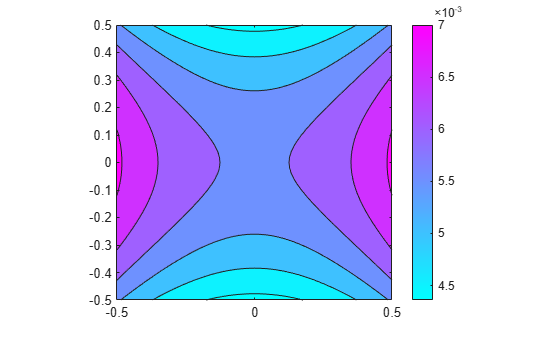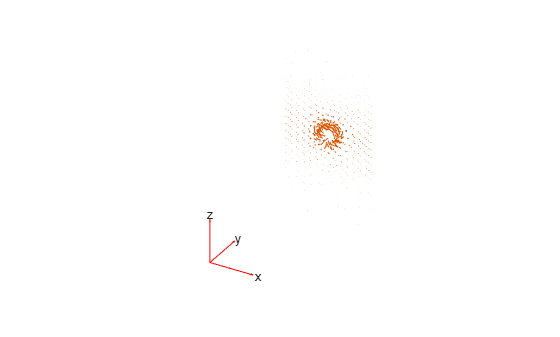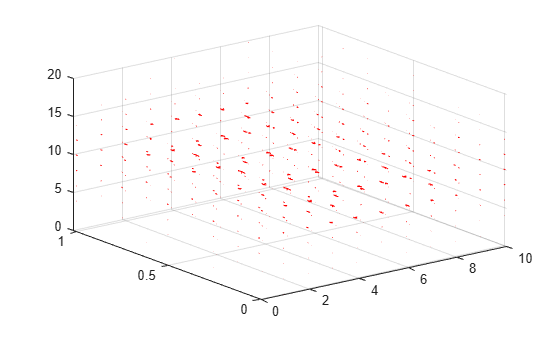# interpolateMagneticPotential

Interpolate magnetic potential in magnetostatic result at arbitrary spatial locations

## Syntax

``Aintrp = interpolateMagneticPotential(magnetostaticresults,xq,yq)``
``Aintrp = interpolateMagneticPotential(magnetostaticresults,xq,yq,zq)``
``Aintrp = interpolateMagneticPotential(magnetostaticresults,querypoints)``

## Description

example

````Aintrp = interpolateMagneticPotential(magnetostaticresults,xq,yq)` returns the interpolated magnetic potential values at the 2-D points specified in `xq` and `yq`. .```

example

````Aintrp = interpolateMagneticPotential(magnetostaticresults,xq,yq,zq)` uses 3-D points specified in `xq`, `yq`, and `zq`.```

example

````Aintrp = interpolateMagneticPotential(magnetostaticresults,querypoints)` returns the interpolated magnetic potential values at the points specified in `querypoints`.```

## Examples

collapse all

Create an electromagnetic model for magnetostatic analysis.

`emagmodel = createpde("electromagnetic","magnetostatic");`

Create a square geometry and include it in the model. Plot the geometry with the edge labels.

```R1 = [3,4,-1,1,1,-1,1,1,-1,-1]'; g = decsg(R1,'R1',('R1')'); geometryFromEdges(emagmodel,g); pdegplot(emagmodel,"EdgeLabels","on") xlim([-1.5 1.5]) axis equal```Specify the vacuum permeability in the SI system of units.

`emagmodel.VacuumPermeability = 1.2566370614E-6;`

Specify the relative permeability of the material.

`electromagneticProperties(emagmodel,"RelativePermeability",5000);`

Apply the magnetic potential boundary conditions on the boundaries of the square.

```electromagneticBC(emagmodel,"MagneticPotential",0,"Edge",[1 3]); electromagneticBC(emagmodel,"MagneticPotential",0.01,"Edge",[2 4]);```

Specify the current density for the entire geometry.

`electromagneticSource(emagmodel,"CurrentDensity",0.5);`

Generate the mesh.

`generateMesh(emagmodel);`

Solve the model and plot the magnetic potential.

```R = solve(emagmodel); pdeplot(emagmodel,"XYData",R.MagneticPotential, ... "Contour","on") axis equal```Interpolate the resulting magnetic potential to a grid covering the central portion of the geometry, for `x` and `y` from `-0.5` to `0.5`.

```v = linspace(-0.5,0.5,51); [X,Y] = meshgrid(v); Aintrp = interpolateMagneticPotential(R,X,Y)```
```Aintrp = 2601×1 0.0056 0.0057 0.0058 0.0059 0.0060 0.0061 0.0062 0.0063 0.0064 0.0065 ⋮ ```

Reshape `Aintrp` and plot the resulting magnetic potential.

```Aintrp = reshape(Aintrp,size(X)); figure contourf(X,Y,Aintrp) colormap(cool) colorbar```Alternatively, you can specify the grid by using a matrix of query points.

```querypoints = [X(:),Y(:)]'; Aintrp = interpolateMagneticPotential(R,querypoints);```

Create an electromagnetic model for magnetostatic analysis.

`emagmodel = createpde("electromagnetic","magnetostatic");`

Import and plot the geometry representing a plate with a hole.

```importGeometry(emagmodel,"PlateHoleSolid.stl"); pdegplot(emagmodel,"FaceLabels","on","FaceAlpha",0.3)```Specify the vacuum permeability value in the SI system of units.

`emagmodel.VacuumPermeability = 1.2566370614E-6;`

Specify the relative permeability of the material.

`electromagneticProperties(emagmodel,"RelativePermeability",5000);`

Specify the current density for the entire geometry.

`electromagneticSource(emagmodel,"CurrentDensity",[0;0;0.5]);`

Apply the magnetic potential boundary conditions on the side faces and the face bordering the hole.

```electromagneticBC(emagmodel,"MagneticPotential",[0;0;0],"Face",3:6); electromagneticBC(emagmodel,"MagneticPotential",[0;0;0.01],"Face",7);```

Generate the linear mesh.

`generateMesh(emagmodel);`

Solve the model.

`R = solve(emagmodel)`
```R = MagnetostaticResults with properties: MagneticPotential: [1x1 FEStruct] MagneticField: [1x1 FEStruct] MagneticFluxDensity: [1x1 FEStruct] Mesh: [1x1 FEMesh] ```

Plot the magnetic potential.

```pdeplot3D(emagmodel,"FlowData",[R.MagneticPotential.Ax ... R.MagneticPotential.Ay ... R.MagneticPotential.Az])```Interpolate the resulting magnetic potential to a grid covering the entire geometry, for `x`, `y`, and `z`.

```x = linspace(0,10,11); y = linspace(0,1,5); z = linspace(0,20,11); [X,Y,Z] = meshgrid(x,y,z); Aintrp = interpolateMagneticPotential(R,X,Y,Z)```
```Aintrp = FEStruct with properties: Ax: [605x1 double] Ay: [605x1 double] Az: [605x1 double] ```

Reshape `Aintrp.Ax`, `Aintrp.Ay`, and `Aintrp.Az` to match the shape of the input grid.

```AintrpX = reshape(Aintrp.Ax,size(X)); AintrpY = reshape(Aintrp.Ay,size(Y)); AintrpZ = reshape(Aintrp.Az,size(Z));```

Plot the resulting magnetic potential.

```figure quiver3(X,Y,Z,AintrpX,AintrpY,AintrpZ,"Color","red")```## Input Arguments

collapse all

Solution of a magnetostatic problem, specified as a `MagnetostaticResults` object. Create `magnetostaticresults` using the `solve` function.

Example: `magnetostaticresults = solve(emagmodel)`

x-coordinate query points, specified as a real array. `interpolateMagneticPotential` evaluates the magnetic potential at the 2-D coordinate points `[xq(i) yq(i)]` or at the 3-D coordinate points `[xq(i) yq(i) zq(i)]` for every `i`. Because of this, `xq`, `yq`, and (if present) `zq` must have the same number of entries.

`interpolateMagneticPotential` converts query points to column vectors `xq(:)`, `yq(:)`, and (if present) `zq(:)`. It returns magnetic potential values as a column vector of the same size. To ensure that the dimensions of the returned solution are consistent with the dimensions of the original query points, use `reshape`. For example, use `Aintrp = reshape(Aintrp,size(xq))`.

Example: `xq = [0.5 0.5 0.75 0.75]`

Data Types: `double`

y-coordinate query points, specified as a real array. `interpolateMagneticPotential` evaluates the magnetic potential at the coordinate points `[xq(i),yq(i)]` for every `i`. Because of this, `xq` and `yq` must have the same number of entries.

`interpolateMagneticPotential` converts query points to column vectors `xq(:)`, `yq(:)`, and (if present) `zq(:)`. It returns magnetic potential values as a column vector of the same size. To ensure that the dimensions of the returned solution are consistent with the dimensions of the original query points, use `reshape`. For example, use `Aintrp = reshape(Aintrp,size(yq))`.

Example: `yq = [1 2 0 0.5]`

Data Types: `double`

z-coordinate query points, specified as a real array. `interpolateMagneticPotential` evaluates the magnetic potential at the 3-D coordinate points `[xq(i) yq(i) zq(i)]`. Therefore, `xq`, `yq`, and `zq` must have the same number of entries.

`interpolateMagneticPotential` converts the query points to column vectors `xq(:)`, `yq(:)`, and `zq(:)`. It returns magnetic potential values as a column vector of the same size. To ensure that the dimensions of the returned solution are consistent with the dimensions of the original query points, use `reshape`. For example, use `Aintrp = reshape(Aintrp,size(zq))`.

Example: `zq = [1 1 0 1.5]`

Data Types: `double`

Query points, specified as a real matrix with either two rows for 2-D geometry or three rows for 3-D geometry. `interpolateMagneticPotential` evaluates the magnetic potential at the coordinate points `querypoints(:,i)` for every `i`, so each column of `querypoints` contains exactly one 2-D or 3-D query point.

Example: For a 2-D geometry, ```querypoints = [0.5 0.5 0.75 0.75; 1 2 0 0.5]```

Data Types: `double`

## Output Arguments

collapse all

Magnetic potential at query points, returned as a vector for a 2-D problem or an `FEStruct` object for a 3-D problem. The properties of `FEStruct` contain the components of the magnetic potential at query points. For query points `i` that are outside the geometry, `Aintrp(i)`, `Aintrp.Ax(i)`, `Aintrp.Ay(i)`, and `Aintrp.Az(i)` are `NaN`. Properties of an `FEStruct` object are read-only.

## Version History

Introduced in R2021a